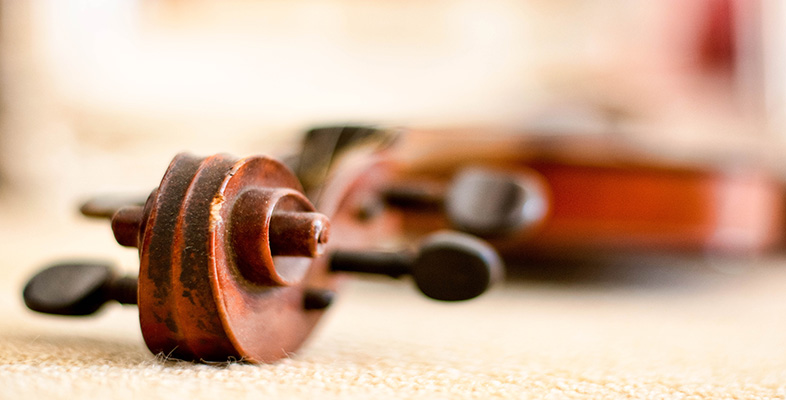Creating musical sounds

This free course is available to start right now. Review the full course description and key learning outcomes and create an account and enrol if you want a free statement of participation.

Free course

# 5.8 Vibrating air column: standing waves in a cylindrical tube open at both ends

The frequencies at which standing waves can be set up in an air column enclosed by a cylindrical tube that is open at both ends can be determined quite easily. Because both ends are open to the atmosphere, the pressure at these positions always remains at atmospheric pressure. In other words, there is no fluctuation in the pressure at the open ends so they must be pressure nodes (think ‘no-deviation’ in pressure). So, as we saw with the string fixed at both ends, the length L of the air column must be equal to an exact number of half wavelengths: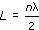where n is an integer number (i.e. 1, 2, 3, 4, …) and λ is the wavelength measured in metres. Following the same arguments as we did for the string, the resonance frequencies of the air column are: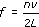where in this case v is the speed of sound in air, which is approximately 340 m/s.

The normal modes of vibration plotted in terms of pressure are shown in Figure 14. For each mode of vibration, I have superimposed a graphical representation of the pressure over a diagram of the air column.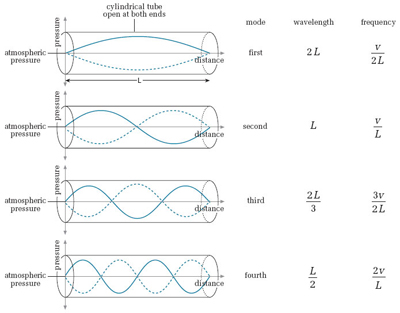Figure 14 The first four normal modes of vibration for a cylindrical tube open at both ends, plotted in terms of pressure. The solid curve shows the pressure at one extreme of the cycle and the dashed curve shows the pressure one half-cycle later

The solid curve shows the pressure at one extreme of the cycle and the dashed curve shows the pressure one half-cycle later. The separation between the curved lines at any position along the tube represents the pressure amplitude at that position.

I've presented these standing-wave patterns in terms of pressure variations in the air column because this is the analysis that will be most useful when we come to describe individual woodwind and brass instruments. However, had we been trying to create an exact analogy with the standing waves on a string we should really have looked at the displacement of the air molecules from the mean position. Thinking in these terms, at the open end of an air column, the air molecules are free to move in and out of the tube. Therefore for an air column open at both ends, the ends must be displacement antinodes. Adjacent antinodes are separated by half a wavelength so the length of the air column must be an integer number of half wavelengths. Displacement nodes are then found at positions halfway between adjacent displacement antinodes.

The normal modes of vibration plotted in terms of displacement are shown in Figure 15. In this case, for each mode of vibration, I have superimposed a graphical representation of the displacement over a diagram of the air column. The solid curve shows the displacement of the air molecules at one extreme of the cycle and the dashed curve shows the displacement one half-cycle later. The separation between the curved lines at any position along the tube represents the displacement amplitude at that position.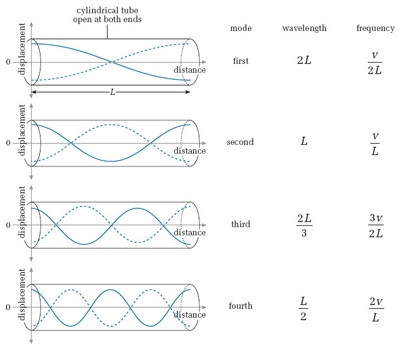Figure 15 The first four normal modes of vibration for a cylindrical tube open at both ends, plotted in terms of displacement. The solid curve shows the displacement at one extreme of the cycle and the dashed curve shows the displacement one half-cycle later

You may well have already realised that pressure nodes correspond to displacement antinodes and that pressure antinodes correspond to displacement nodes. If not, compare the positions of the nodes and antinodes in Figures 14 and 15 to satisfy yourself that this is the case.

## Activity 17

Run the Flash animation below. This animation demonstrates standing waves in a cylindrical tube open at both ends. It shows graphical representations of both the pressure variation along the tube and the displacement of the air molecules from their mean position. This should help to clarify the relationship between these two quantities. It also shows the motion of the air molecules.

Use the controls below (Play/Pause, Step, Reset) to run flash animation. You can also select the mode.

Active content not displayed. This content requires JavaScript to be enabled, and a recent version of Flash Player to be installed and enabled.
Interactive feature not available in single page view (see it in standard view).

Just as was the case for the string fixed at both ends, the frequencies at which standing waves are set up in a cylindrical air column with two open ends form a harmonic series. The first resonance frequency (the fundamental frequency of the air column) is v/2L. If this frequency is denoted f1, then the frequencies at which the second, third, fourth and later modes of vibration occur are 2f1, 3f1, 4f1 …, nf1.

## Activity 18

Calculate the first three resonance frequencies of a 1.5 m long cylindrical tube open at each end. Assume the speed of sound is 340 m/s.

### Answer

If the cylinder is open at both ends, then the formula that gives the resonance frequencies is:where L is the length of the cylinder and v is the speed of sound.

If L = 1.5 m and v = 340 m/s, the first three resonance frequencies are determined as follows:

First resonance frequency f1 = v/2L = 340/(2 × 1.5) = 113.3 Hz.

Second resonance frequency f2 = 2v/2L = (2 × 340)/(2 × 1.5) = 226.7 Hz.

Third resonance frequency f3 = 3v/2L = (3 × 340)/(2 × 1.5) = 340 Hz.

TA212_2

### Take your learning further

Making the decision to study can be a big step, which is why you'll want a trusted University. The Open University has 50 years’ experience delivering flexible learning and 170,000 students are studying with us right now. Take a look at all Open University courses.

If you are new to university level study, find out more about the types of qualifications we offer, including our entry level Access courses and Certificates.

Not ready for University study then browse over 900 free courses on OpenLearn and sign up to our newsletter to hear about new free courses as they are released.

Every year, thousands of students decide to study with The Open University. With over 120 qualifications, we’ve got the right course for you.

Request an Open University prospectus